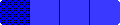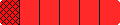Home

# Grades 5-8 Fraction Bars Teacher's Guide

Also See: Standards for Grades 3 to 4
 NCTM STANDARDS 2000 ON TEACHING FRACTIONSEach of the following files has a statement from NCTM's Standards and a corresponding page from the Grades 5-8 Teacher's Guide that illustrates the NCTM statement. Equality - comparing shaded amounts Equality - creating equivalent fractions Inequality - comparing fractions to 1/2 Inequality - comparing fractions Addition - adding fractions with common denominators Addition - adding fractions Subtraction - subtracting mixed numbers Multiplication - fractions times whole numbers Division - dividing fractions by fractions

width="100%"width="100%"